# 2nd PUC Basic Maths Question Bank Chapter 9 Stocks and Shares Ex 9.1

Students can Download Basic Maths Exercise 9.1 Questions and Answers, Notes Pdf, 2nd PUC Basic Maths Question Bank with Answers helps you to revise the complete Karnataka State Board Syllabus and score more marks in your examinations.

## Karnataka 2nd PUC Basic Maths Question Bank Chapter 9 Stocks and Shares Ex 9.1

Part – A

2nd PUC Basic Maths Stocks and Shares Ex 9.1 One Mark Questions with Answers ( 1 ×  10 = 10 )

Question 1.
Find the income obtained by investing 3600 in 5% stock at 90.Question 2.
How much stock at ₹ 75 can be bought for ₹ 3375.
Amount 75 – Stock 100
Amount 3375 → $$\frac{3,375 \times 100}{75}$$ = 4,500
∴ Stock = ₹ 4,500

Question 3.
6% stock is being sold at 15 discount. How much money is required to buy ₹ 6000 stock.
Amount 85 – Stock 100
Amount? Stock 6,000
∴ Amount = $$\frac{6,000 \times 85}{100}$$ = 5,100Question 4.
Define yield.
Yield is defined as nominal interest divided by Amount invested

Question 5.
What is the yield obtained when ₹ 5000, 3% stock is purchased at 125.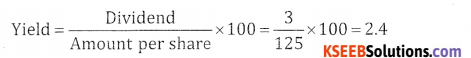Question 6.
What income can be obtained from an investment of ₹ 10,725 in 6.5% stock at 143. What is the amount of stock purchased?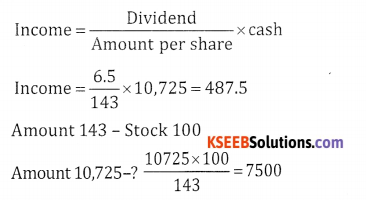Question 7.
10% stock is quoted at ₹ 120. Find the rate of interest.
[Hint: here rate of interest means yield]
Here rate of interest means yield]
Interest = $$=\frac{D}{A}$$ X 100
= $$=\frac{10}{120}$$ x 100 = 8.33%Question 8.
How much of 8% stock at 96 can be purchased for ₹ 4800? Also find the income obtained?
Income = $$=\frac{D}{A}$$ X Cash
Income = $$=\frac{8}{96}$$ X 4800
Income = ₹ 400

Question 9.
How much money will a man get by selling ₹ 6000 at 5% stock at 20 premium?
Stock 100 – Amount 120
Stock 6000 – ?
Amount = $$\frac{6,000 \times 120}{100}$$ = ₹ 7,200

Question 10.
Abhi purchased 850 shares of a company the nominal value being 10 each. Company declared an annual
dividend of 12%. How much dividend did abhi receive?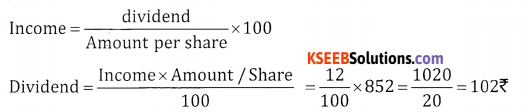Part – B

2nd PUC Basic Maths Stocks and Shares Ex 9.1 Three or Four Marks Questions with Answers

Question 1.
Rakshitha invests ₹ 15000 in a company paying 7% per arum, when a share of value ₹ 100 is selling for ₹ 150. What is her annual income and what percentage does she get on her money?
Income = $$\frac{\text { Dividend }}{\text { Amount }} \times Cash$$
= $$\frac{7}{150}$$x 1500 = ₹ 700

Question 2.
Which is a better investment?
(a) 8% stock at 110 = $$\frac{8}{110}$$ × 100 = 7.272%
9% stock at 98 = $$\frac{9}{98}$$ × 100 = 9.18%
98 9.18% > 7.272
∴ 9% stock at 98 is a better investment

(b) 6 $$\frac{1}{2}$$ % stock at 84 $$\frac{\frac{13}{2}}{\frac{84}{84}}$$ × 100 = 5.9%
4% stock at 102 = $$\frac{4}{102}$$ × 100 = 3.92%
∴ 6$$\frac{1}{2}$$ % stock at 84 is a better investment

(C) 7% stock at 115 = $$\frac{7}{115}$$ × 100 = 6.08%
5% stock at 88 = $$\frac{5}{88}$$ × 100 = 5.68%
Here 7% stock at 115 is better investment

(d) 4% stock at 118 =
$$\frac{4}{118}$$ × 100 = 3.38%
6% stock at 124 = $$\frac{6}{124}$$ × 100 = 6.83%
∴ 6% stock at 124 is better investment

Question 3.
A man invests equal sums of money in 4%, 5% and 6% stock, each stock being at par. If the total income of the man is 23,600. Find his total investment.
Let the amount invested be x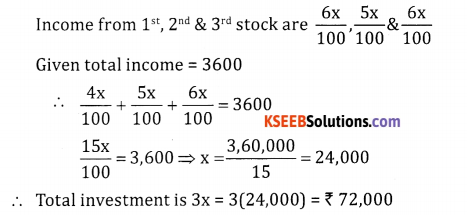Question 4.
What is the market value of 9.5% stock when an investment of ₹ 12,400 produces an income of ₹1472.5?
Income = $$\frac{\text { Dividend }}{\text { Amount pershare }} \times cash$$
1472.5 = $$\frac{9.5}{\mathrm{A}}$$ × 12,400
⇒ A = $$\frac{9.5 \times 12,400}{1972.5}$$ = ₹ 80
Market value per share = ₹ 80

Question 5.
What is the quoted value of 12% stock if it earns an interest of 8% after deducting the income tax of 8%.
Net income 92 – open income is 100
Net income 8 – ? $$\frac{100 \times 8}{92}$$ , Dividend = 12
Income = $$\frac{D}{A}$$ x cash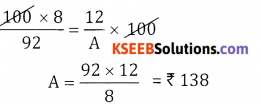Question 6.
Venu invested ₹ 5,02,950 in Infosys when price of ₹5 shares was 3353. He sold shares worth ₹ 500 when the price went upto 3583 and the remaining he sold when the price was ₹ 3253. How much did Venu gain.
Number of shares = $$\frac{50,2950}{3,353}$$ = 150
∴ Stock = 5 x 150 = 750
Stock work 500 means 100 shares
Price of 1 share is 3,585
Price of 100 shares = 3,58,300
Remaining shares = 50
Price of 1 share is 3,253
Price of 50 shares = 1,62,650
∴Total amount received after selling the share’s is 3,58,300 + 1,62,650 = ₹ 5,20,950
Hence venu gaind 5,20,950 – 5,02,950 = ₹ 18,000Question 7.
Ramesh has invested ₹ 4,300 partly in 4.5% stock at ₹ 72 and partly in 5% stock at ₹ 95. If the total income
from both is ₹ 250, find the investment in both the types of stock.
Let the amount invested in 4.5% stock be x
Let the amount invested in 5% stock be 4,300 – x
45 Income obtained from 4.5% stock is $$\frac{4.5}{72}$$ × x
Income obtained from 5% stock is $$\frac{5}{95}$$ × (4,300 – x)
Total interest income from both = 250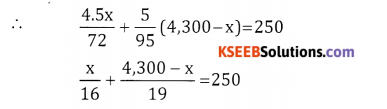19x + 16 (4,300 – x) = 250 R 16 × 19
3x = 16 (4,750 – 4,300) ⇒ 3x = 16 × 450 ⇒ x = 2,400
The amount invested in 4.5% stock is ₹ 2,400
The amount invested in 5% stock is ₹ 1,900

Question 8.
Ramesh holds ₹ 2,100 of 3% stock. He sells at
₹ 121 and invests the proceeds in 5% stock. Thereby his income increases by 14. Find the market price of 5% stock.
Stock 100 – amount 121
Stock 2100 – ?
Amount = $$\frac{2,100 \times 121}{100}$$ = 2,541
Income = $$\frac{D}{A}$$ × 100
77 = $$\frac{8}{A}$$ x 2,541
Amount = $$\frac{5 \times 2,541}{77}$$ = ₹ 165
Market price of 5% stock = ₹ 165

Question 9.
How much must be invested in 14.25% stock at 98 to produce the same income as would be obtained by investing ₹ 9,975 in 15% stock at 105.
Let the cash invested be x
Income from 14.25% stock at 98 = $$\frac{14.25}{98}$$ x x
Income from 15% stock at 105 by investing
₹ 9,975 = $$\frac{15}{105}$$ × 9,975
Given both the incomes are equal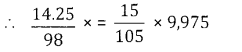⇒ x = 9,800
∴ Amount to be invested = ₹ 9800

Question 10.
A stock yields 5% to an investor. A fall of 75 in its price causes it to yield 5 42%. What was its market value if the two income are equal.
Let dividend I = D1 = 5,& D2 = 5 $$\frac{1}{2}$$
& Amount per share ₹ x & ₹ x-5
Incomes are equal i.e. $$\frac{D_{i}}{A_{1}}=\frac{D_{2}}{A_{2}}$$
$$\frac{5}{x-5}=\frac{11}{2 x}$$
10x = 11x – 55; 55 = 11x – 10x = x in
∴ its market value is 55.

Question 11.
Sanjana invests ₹ 3240 in a stock at 108 and sells when the price falls to 104. How much stock at 130 can Sanjana now buy.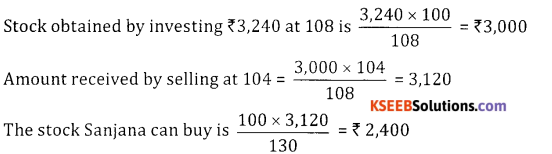Question 12.
A person invested 4200 partly in 5% stock at 125 and the remaining in 7.5% stock at 75. If income derived
from the two stocks is the same. Find the respective investments in each stock. Also find the total income
Let ₹ x be invested in 5 % stock at 125 & ₹(4,200 – x) will be invested in 7.5% at 75
I1 = $$\frac{5}{125}$$ × x
I2 = $$\frac{7.5}{75}$$ × (4,200 – x)
Both incomes are equal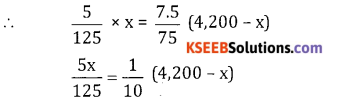50x = 5,25,000 – 125x;
175x = 5,25,000 ⇒ x= 3,000
Amount invested in 5% stock is ₹ 3,000 & 7.5% is ₹ 1,200
Income from I stock = $$\frac{5}{125}$$ ⇒ x 3,000 =120 & Total income ⇒ 120 + 120 = 240

Question 13.
If a person wishes to obtain 18% yield from his investment at what price should he buy 13.5% stock.
Given yield = 18%, D = 13.5, A = ?
Yield = $$\frac{\mathrm{D}}{\mathrm{A}}$$ × 100
18 = $$\frac{13.5}{A}$$ x 100 ⇒ A = $$\frac{13.5 \times 100}{18}$$ ₹ 75Question 14.
(a) A invests a sum of money in 5.5% stock at 90 and B and equal sum in 3.5% stock. If A’s income is
10% more than B’s find the price of 3.5% stock.
(b) How much money has to be invested in 11.5% stock at 73 to obtain an income of ₹ 150 after a tax deduction at source of 20%.
(a) A9 income = $$\frac{55}{90}$$ × x
B9 income = $$\frac{3.5}{y}$$ × x
Where x = investment
y = price of 3.5% stock
A9 income: B19 income :: 110 : 100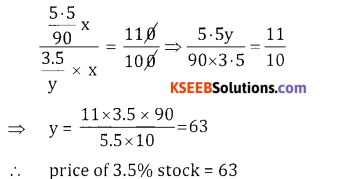(b)
net income is 150
tax deduction at 20%
Net income 80 – gross income 100
If net income is 150 – ?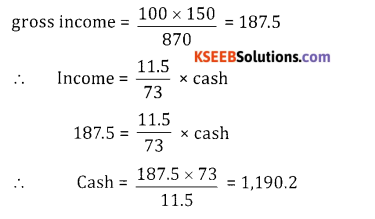Question 15.
(a) A person invests ₹15000 partly in 3% stock at 75 and partly in 6% stock at 125. If the income from
both is ₹ 675 find his investment in the 2 types of stock.
(b) At what price a person has bought 25% shares with face value 100 which has give him 20% returns.
(a) Let x be invested in I stock & (15,000 – x) will be invested in II stock.
I1 = Income obtained from Ist stock is $$\frac{3 x}{75}$$
I2 = Income obtained from 2nd stock = $$\frac{6}{125}$$ (15,000-x)
Given Total income = I1 + I2 = 675
$$\frac{3 x}{75}$$ + $$\frac{6}{125}$$ (15,000-x) = 675
0.008 x = 45 ⇒ x= 5,625
∴ Amount invested in 1st stock is ₹ 5,625 and in 2nd stock is ₹ 9,375

(b) Yield = 20%, dividend = 25%
Yield = $$\frac{\mathrm{D}}{\mathrm{A}}$$ = x 100
20 = $$\frac{25} {\mathrm{A}}$$ × 100
Α = $$\frac{25 \times 100}{20}$$ = 125Question 16.
Rohan invested ₹ 55000 partly in 8% stock at 80 and partly in 12% stock at 150 in such a way as to get a
return of 9% for his money. How much did Rohan invest in each.
Let ₹ x be invested in 8% stock at 80 & ₹ (55,000 – x) be invested in 12% stock at – 150.
Total income = $$\frac{9}{100}$$ × 55,000 = 4,950
Income in I stock = $$\frac{8}{800}$$ x
Income in II stock = $$\frac{12}{150}$$ (55,000 – x)
Total income = 4,950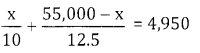12.5 x + 5,50,000 – 10x = 4,950 × 10 × 12.5
2.5x = 61,87,500 – 5,50,000
2.5x = 68,750 ⇒ x= 27,500
Rohan invests 27,500 in each

Question 17.
Prathik sells out ₹ 6000 of 7.5% stock at 108 and reinvests the proceeds in 9% stock. If Prathik’s income
increases by 270. At what price did Prathik buy 9% stock.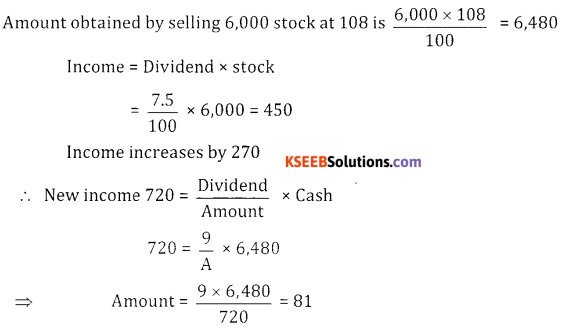Question 18.
Jane sells her ₹ 12500, 4.5% stock at 94. How much of 9% stock at 125 can Jane purchase from the sale
proceeds of the former stock. What is the change in Jane’s income.
Amount obtained by selling 1,25,000 stock at 94 is $$\frac{12,500 \times 94}{100}$$ = ₹ 11.750
100 Jane 13 income from 4.5% stock is
= $$\frac{45}{100}$$ × 12,500 562.5
11,750 x 100 9% stock purchased at 125 is = $$\frac{11,750 \times 100}{125}$$ = 9,400
125 Income from 9% stock is , $$\frac{9}{100}$$ × 9400 = 846
100 Change in income = 846 – 562.5 = ₹ 283.5

Question 19.
Aman sells ₹ 25000, 13.5% stock when the shares were selling at a premium of 20. He invests the proceeds
partly in 15% stock at 75 and partly in 16% stock at 128. Find how much he has invested in each stock if
his income increases by ₹ 1875.
Amount obtained by Selling 25,000
Stock at ₹ 120 is $$\frac{25,000 \times 120}{100}$$ = 30.000
100 Let ₹ x be invested in 15% stock at 75
& ₹ (30,000 – x) invested in 16% stock at 128
Income from 25,000 stock is $$\frac{13.5}{100}$$ × 25,000 = 3,375
100 New income = 3,375 + 1,875 = 5,250
Income from 15% stock at 75 = $$\frac{15 x}{75}$$
Income from 16% stock at 128 = $$\frac{16}{128}$$ (30,000 – x)
Total income = 5,250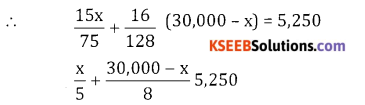8x + 1,50,000 – 5x = 5,250 × 40
3x = 60,000 ⇒ x = 20,000
∴ He invested 20,000 at 15% & 10,000 at 16%Question 20.
Nihal has ₹ 5 Maruthi shares worth ₹ 1000. He sells 120 shares when the shares are selling at ₹ 1400 and
the remaining shares when the price goes upto ₹ 1600. He invests the proceeds in ₹ 10 Maruthi shares selling at ₹ 1184 find the number of Maruthi shares purchased by Nihal.
No. of Shares = $$\frac{1,000}{5}$$ = 200
Amount received by selling 120 shares at 1400 = 120 × 1,400 = 1,68,000
Amount received by selling 80 shares art 1,600 = 80 × 1,600 = 1,28,000
∴ Total amount = 1,68,000 + 1,28,000 = 2,96,000
∴ Number of shares purchased = $$\frac{29,6000}{1,184}$$ = 250

Question 21.
A man owns 50 SBI shares which are now selling at the rate of 1800. He needs 50,000 for his daughter’s
education. He decides to sell 25 SBI shares. The brokerage charged is 25%. How much more money does
he need to arrange after selling the share.
Amount received after selling 25 shares at ₹ 1800 = 45,000
Brokerage = $$\frac{25}{100}$$ × 45,000 = 112.5
Money obtained = 45,000 – 112.5 = 4,4887.5
He has to arrange for 50,000 – 4,4887.5 = 5112.5

Question 22.
Rakshith decides to invest in TCS shares which are selling at 2020 per share. How much money is required
to purchase 10 shares if the brokerage is 0.5%.
Selling price of 10 shares at 2020 per share = 20,200
0.5 Brokerage = $$\frac{0.5}{100}$$ × 20,200 = ₹ 101
100 Amount required to purchase = 20,200 + 101 = 20,301

Question 23.
Ritu purchased 200 HDFC shares when the price was 625 and then sold all the shares when the price
went upto 715. If the brokerage for each transaction was 1%. How much did Ritu gain.
Selling price of 200 shares at 625 = 1,25,000
Brokerage is $$\frac{1}{100}$$ × 1,25,000 = 1,250
Amount paid = 1,25,000 + 1,250 = 1,26,250.
Selling price of 200 shares at 715 = 1,43,000
Brokerage is $$\frac{1}{100}$$ × 1,43,000 = 1,430
Amount received = 1,43,000 – 1,430 = 1,41,570
Ritu gained = 1,41,570 – 1,26,250 = ₹ 15,320

Question 24.
If a client buys shares worth ₹90,000 and sells shares worth ₹ 1,10,000 through a stock broker calculate
the brokerage payable to the stock broker if the brokerage rate is 0.5% each side.
Brokerage is $$\frac{0.5}{100}$$ × (1,10,000 – 90,000) = $$\frac{0.5}{100}$$ × 20,000 = ₹ 100

Question 25.
Veena buys 100 shares of Karnataka bank at ₹ 101 per share. She pays ₹ 10,130.3 to her broker. What is
the total brokerage she paid and calculate the percentage rate of brokerage.
Selling price of 100 shares at 101 = 10,100
Amount paid is 10130.3
Brokerage is 10130.3 – 10,100 = 30.3
100 x 30.3 Rate of brokerage is $$\frac{100 \times 30.3}{10,100}$$ = 0.3%Question 26.
Mr. Ravi sold ₹ 2,250 stock at 75 and bought stock at 88.5 with the proceeds. How much stock does he buy
if the brokerage is 2% for selling and 1.5% for buying.
Mr. Ravi selling price of 2,250 stock at 75 is = $$\frac{2,250 \times 75}{100}$$ = 1,687.5
Brokerage is $$\frac{2}{100}$$ × 1,687.5 = 33.75
Brokerage is on cash = $$\frac{1,653.75 \times 1.5}{100}$$ = 24.81
Stock purchased at 88.5 = $$\frac{1,628.94 \times 100}{88.5}$$ = 1,840.61